# Python Program to Interchange Digits of a Number

• Interchange the first and last digits of a given number.
• Interchange the digits of a given number using a list.
• Interchange any two desired digits of a given number.

## Interchange the first and last digits of a number

The question is: write a Python program to interchange the first and last digits of a number given by the user. Here is its answer:

```print("Enter the Number: ")
num = int(input())
rev = 0
noOfDigit = 0
temp = num
while temp>0:
temp = int(temp/10)
noOfDigit = noOfDigit+1
if noOfDigit<2:
print("\nIt is a Single-digit Number!")
elif noOfDigit==2:
temp = num
while temp>0:
rem = temp%10
rev = (rev*10)+rem
temp = int(temp/10)
print("\nFirst and Last (Second) Digit Interchanged Successfully!")
print("\nNew Number: ")
print(rev)
else:
temp = num
while temp>0:
rem = temp%10
rev = (rev*10)+rem
temp = int(temp/10)
revNum = rev
rev = 0
temp = num
noOfDigitTemp = noOfDigit
while temp>0:
remTemp = revNum%10
if noOfDigitTemp==noOfDigit:
rem = temp%10
rev = (rev*10)+rem
elif noOfDigitTemp==1:
rem = temp%10
rev = (rev*10)+rem
else:
rev = (rev*10)+remTemp
temp = int(temp/10)
revNum = int(revNum/10)
noOfDigitTemp = noOfDigitTemp-1
print("\nFirst and Last Digit Interchanged Successfully!")
print("\nNew Number: ")
print(rev)```

Here is its sample run: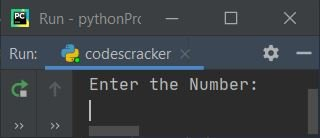Now supply the input, say 12034, as a number to interchange the first (1) and last (4) digits of the given number: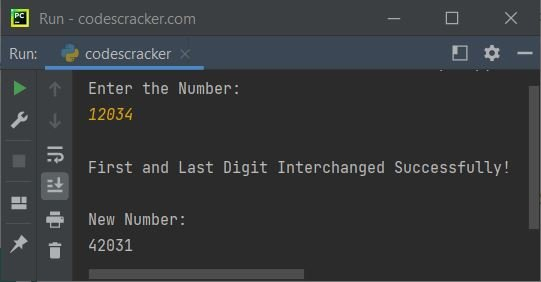Here is another sample run with user input: 12.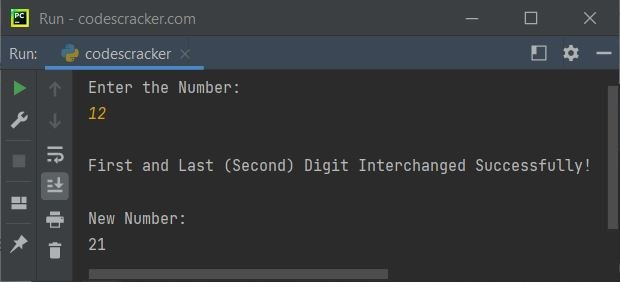## Interchange the first and last digits using a list

This program is similar to the previous one. The only difference is that this program uses a Python list to do the job.

```print(end="Enter the Number: ")
num = int(input())
rev = 0
noOfDigit = 0
temp = num
while temp>0:
temp = int(temp/10)
noOfDigit = noOfDigit+1
temp = num
while temp>0:
rem = temp%10
rev = (rev*10)+rem
temp = int(temp/10)
nums = []
for i in range(noOfDigit):
rem = rev%10
nums.insert(i, rem)
rev = int(rev/10)
if noOfDigit==1:
print("\nIt's a single-digit number!")
elif noOfDigit==2:
temp = nums
nums = nums
nums = temp
print("\nFirst and Last (Second) Digit Interchanged Successfully!")
print(end="The New Number = " +str(nums)+str(nums))
else:
i = 0
temp = nums[i]
nums[i] = nums[noOfDigit-1]
nums[noOfDigit-1] = temp
print("\nFirst and Last Digit Interchanged Successfully!")
print(end="The New Number = ")
for i in range(noOfDigit):
print(end=str(nums[i]))```

Here is its sample run with user input, 230498: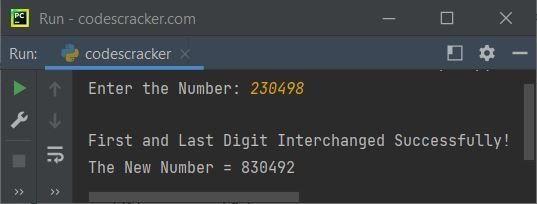## Interchange any two desired digits of a number

The above two programs interchange the first and last digits of a given number automatically. But what if the user wants to interchange any two particular digits of a number entered by him or her?

To overcome this problem, we have another program that interchanges any two required digits of a given number. Here is the program:

```print(end="Enter the Number: ")
num = int(input())
rev = 0
noOfDigit = 0
temp = num
while temp>0:
temp = int(temp/10)
noOfDigit = noOfDigit+1
if noOfDigit==1:
print("\nIt's a single-digit number!")
else:
print(end="\nInterchange the Digit at Position: ")
posFirst = int(input())
print(end="With Digit at Position: ")
posSecond = int(input())
if posFirst>noOfDigit or posSecond>noOfDigit:
print("\nInvalid Input!")
else:
temp = num
while temp>0:
rem = temp%10
rev = (rev*10)+rem
temp = int(temp/10)
arr = []
for i in range(noOfDigit):
rem = rev%10
arr.insert(i, rem)
rev = int(rev/10)
i = 0
temp = arr[posFirst-1]
arr[posFirst-1] = arr[posSecond-1]
arr[posSecond-1] = temp
print("\nDigits Interchanged Successfully!")
print(end="The New Number = ")
for i in range(noOfDigit):
print(end=str(arr[i]))```

Here is its sample run with user input: 1234 as a number, 1 as the position of the first digit to interchange with the digit at position 2: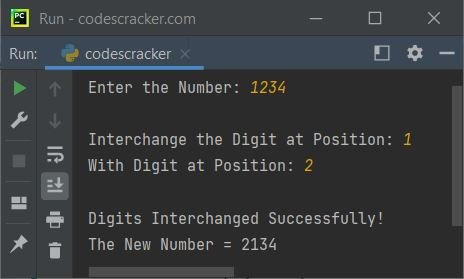Python Online Test

« Previous Program Next Program »